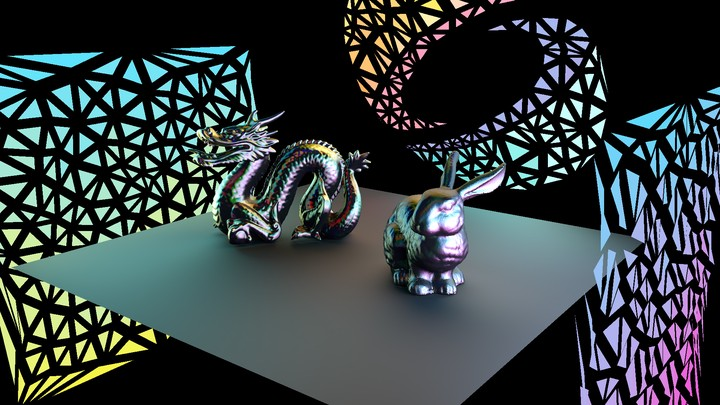Analytic Spherical Harmonic Gradients for Real-Time Rendering with Many Polygonal Area LightsAbstract

Recent work has developed analytic formulae for spherical harmonic (SH) coefficients from uniform polygonal lights, enabling near-field area lights to be included in Precomputed Radiance Transfer (PRT) systems, and in offline rendering. However, the method is inefficient since coefficients need to be recomputed at each vertex or shading point, for each light, even though the SH coefficients vary smoothly in space. The complexity scales linearly with the number of lights, making many-light rendering difficult. In this paper, we develop a novel analytic formula for the spatial gradients of the spherical harmonic coefficients for uniform polygonal area lights. The result is a significant generalization, involving the Reynolds transport theorem to reduce the problem to a boundary integral for which we derive a new analytic formula, showing how to reduce a key term to an earlier recurrence for SH coefficients. The implementation requires only minor additions to existing code for SH coefficients. The results also hold implications for recent efforts on differentiable rendering. We show that SH gradients enable very sparse spatial sampling, followed by accurate Hermite interpolation. This enables scaling PRT to hundreds of area lights with minimal overhead and real-time frame rates. Moreover, the SH gradient formula is a new mathematical result that potentially enables many other graphics applications.

Publication
ACM Transactions on Graphics (SIGGRAPH 2020), 39(4), July 2020Guangyan Cai
Ph.D. Student in Computer Science

My research interests include physics-based rendering and differentiable rendering.